# Complementary angles

Two angles are complementary. If one angle measures 10°, what is the measure of the second angle?

Correct result:

x =  80 °

#### Solution:

$x=9{0}^{\circ }-1{0}^{\circ }=8{0}^{\circ }$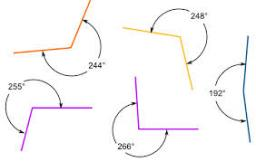We would be pleased if you find an error in the word problem, spelling mistakes, or inaccuracies and send it to us. Thank you!## Next similar math problems:

• Internal and external anglesCalculate the remaining internal and external angles of a triangle, if you know the internal angle γ (gamma) = 34 degrees and one external angle is 78 degrees and 40 '. Determine what kind of triangle it is from the size of its angles.
• It is rectangular?Size of two of the angles in a triangle are: α=110°, β=40°. Is it a right triangle?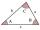The size of two internal angles of a triangle ABC are α=6/18π and β=7/18π. Calculate the size of the third angle.
• Cost reductionTwo MP3 players whose price was equal to originally have been discounted the first by 20%, the second by 35%. After the price reduction was the difference in their prices 750, - CZK. What was the original price of each of the two players?
• Two ribbonsThe total length of the two ribbons is 13 meters. If one ribbon is 7 and 5/8 meters long, what is the length of the other ribbon?
• OpheliaOphelia recorded the temperature of a cold store every two hours. 1. At 6am it was -4°C and at 8am it was -1°C. By how much did the temperature rise? 2. The temperature went up by 5°C in the next two hours. .What was the temperature at 10am?
• Difference in temperaturesThe temperature in the number line show minus 15 degree celsius and 8 degree celsius. What is the different between the temperatures?
• Clock Tower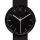What angle is betwenn hands on Clock Tower when show 17 hours and 35 minutes?
• Two gardenersThe garden with an area of 81 square meters was divided by two gardeners in a ratio of 4: 5. How much did the second gardener get more first?
• Scientific notation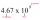Approximately 7.5x105 gallons of water flow over a waterfall each second. There are 8.6x104 seconds in 1 day. Select the approximate number of gallons of water that flow over the waterfall in 1 day.
• Flowerbed 2Around the square flower bed in a park is sidewalk 2 m wide. The area of ​​this sidewalk is 243 m2. What is the area of the flowerbed?
• Angles in triangle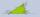Calculate the alpha angle in the triangle if beta is 61 degrees and 98 gamma degrees.
• TrianglesFind out whether given sizes of the angles can be interior angles of a triangle: a) 23°10',84°30',72°20' b) 90°,41°33',48°37' c) 14°51',90°,75°49' d) 58°58',59°59',60°3'
• Bus vs. trainBus started from point A 10 minutes before the train started from the same place. The bus went an average speed of 49 km/h, train 77 km/h. To point B train and bus arrived simultaneously. Calculate time of train journey, if train and bus travelled the sam
• Christmas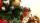Exactly after 114 hours we sit down to Christmas Eve table. What day and what time it was when Dad said this sentence. The Christmas Eve table they sit down exactly on the 18-o'clock (6 PM).
• Square and circle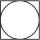Into square is inscribed circle with diameter 10 cm.What is difference between circumference square and circle?
• LaboratoryMang Elio went to his laboratory at 7:00am And recorded that the temperature was 11.07°C. At lunchtime, the temperature rose by 27.9°C but dropped by 8.05°C at 5:00 PM. What was the temperature at 5:00 PM?